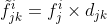# Multiplying Tensors

I have to perform the operation:I have two tensors that I want to multiply in this way, `A` and `B`:

``````A = torch.randn(B,C,j,i)
B = torch.randn(B,C,j,k)
C = AxB
C.shape = torch.Size(B,C,j,i,k)
``````

Where I have assumed the shape of the output tensor based on the indices of the equation.

What is the function that I should use to produce the desired result?

Would this code work?

``````B, C, i, j, k = 2, 3, 4, 5, 6

A = torch.randn(B,C,j,i)
B = torch.randn(B,C,j,k)

C = torch.matmul(A.unsqueeze(4), B.unsqueeze(3))
print(C.shape)
``````